## Section32.3Electric Field Inside Conductors

Vanishing of Electric Field Inside Conductors

In Section 32.2 we saw that when you bring an electric charge near a metal the conduction electrons in the metal respond to the electric field of the charge and move from one region to another region leading to the creation of positive and negative sides on the metal surface. The material in the volume of the metal, however, remains neutral containing a large number of conduction electrons that balance the charges of the protons in the nuclei in the volume of the material.

When the movement of the electrons stops, the force on the conduction electrons, both at the surface and in the volume, must be balanced otherwise the conduction electrons will continue to move. Therefore, we conclude that the net electric field inside metals must be zero when the static condition has reached.

\begin{equation} \vec E_P = 0\ \ \ (\text{Static; P inside conductor.})\tag{32.3.1} \end{equation}

How does the vanishing of the electric field inside the conductor occur? Let us look at as simple example of a point charge $q$ near a neutral metal sphere as shown in Figure 32.3.1.

We have three sources of electric field and the net electric field is a vector sum of the fields of $+q\text{,}$ and the surface charge densities $-\sigma_A$ and $+\sigma_B$ on the metal due to its polarization.

That is, the net field at P will be different from what it was in the absence of the metal.

\begin{equation} \vec E_{\text{net}} = \vec E_{\text{of }q} + \vec E_{\text{of }\sigma_A} + \vec E_{\text{of }\sigma_B}.\tag{32.3.2} \end{equation}

As you bring charge $q$ near the metal, net field at P may not vanish initially, leading to migration of charges, but when static equilibrium has reached, the charge densities of the induced charges will be such that electric field inside the conductor must have vanished. Otherwise, we will still have moving charges inside the conductor, and by definition, that is not the case in the static equilibrium.

\begin{equation} \vec E_{\text{net}} = 0\ \ \ (\text{at points inside the conductor})\tag{32.3.3} \end{equation}

Vanishing of Electric Field Inside Conductor and Charging a Conductor

The vanishing of electric field inside a conductor also has implications on the charging of a conductor. Suppose you put some extra electrons, say a total charge, $-Ne\text{,}$ anywhere in a conductor. The new charges placed in a conductor will disturb the balance that existed before, and the conduction electrons in the conductor will rearrange themselves. One can ask the following question: after the static condition has reached again, where will be the charges? The general rule is that the charges will redistribute so that the electric field at space points that are inside the conductor will be zero when the equilibrium has reached again.

This is required for the consistency of our arguments. If there were any net charge in a volume element of the conductor, electric field in its neighborhood will not be zero as can be easily seen by either drawing the electric field lines of the net charge, or by using the Gauss's law with a Gaussian surface enclosing the volume element that has a net charge (Figure 32.3.2).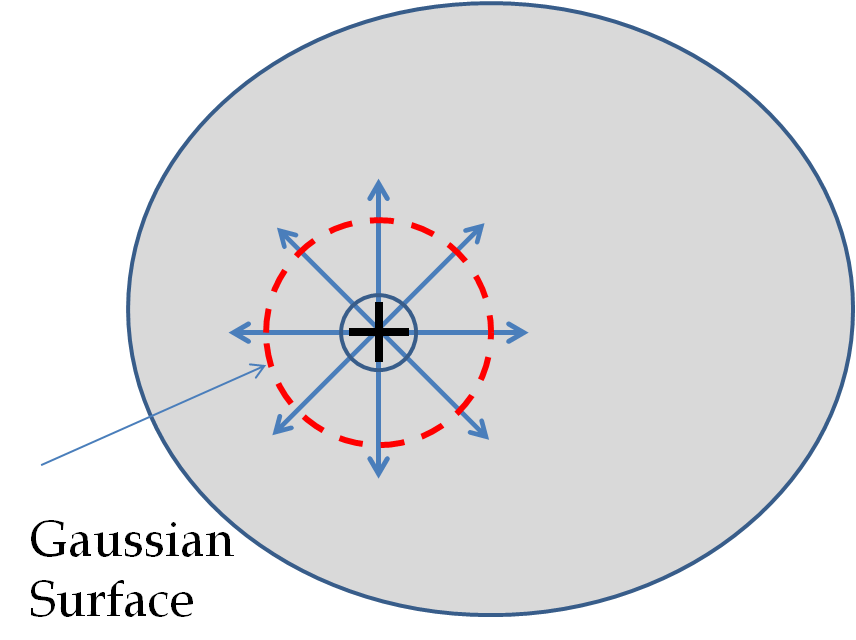Figure 32.3.2. The electric field lines of a net positive charge inside a volume point of a conductor will give a net electric flux non-zero. But since, electric field is zero inside, a calculation of electric flux from electric field will be zero. These two values of electric flux contradict each other.

If the Gaussian surface encloses any net charge, the flux through it will be non-zero.

\begin{equation} \Phi_E = \frac{q}{\epsilon_0} \ \ \Longrightarrow \Phi_E \ne 0 \ \ \Longrightarrow \vec E \ne 0.\tag{32.3.4} \end{equation}

A non-zero electric field inside the conductor will cause the acceleration of free charges in the conductor, violating the premise that the charges are not moving inside the conductor. Hence, we conclude that any excess charge put inside an isolated conductor will end up at the boundary surface when the static condition has reached.

Electric Field Shielding

One can take advantage of the vanishing of electric field inside a conductor and construct a metal cage, called the Faraday cage, to screen out electric field from entering an enclosed space. One does not even need to cover all the space; usually a metal screen is sufficient to shield from the external electric field.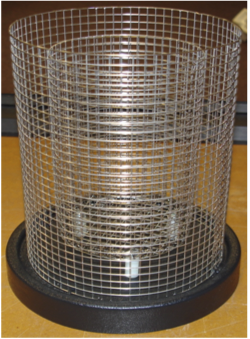Is Electric Field Inside a Metal Always Zero?

The argument for the vanishing of the electric field inside a metal required static conditions on the conduction electrons. When a metal wire carries current the conduction electrons are not static. Therefore, electric field will not be zero inside a metal that is carrying a current.

Note that often-quoted simplistic rule that, “the electric field inside a conductor is zero,” applies only to static situations. If electric field were zero in all situations, then there will be no electric current in a metal wire. The conduction of electricity requires non-zero electric field inside a conductor.

(Calculus) Conductors as Equipotential Space

The vanishing of electric field inside a conductor also means that electric potential inside the conductor is constant as the following calculation shows ( Figure 32.3.3).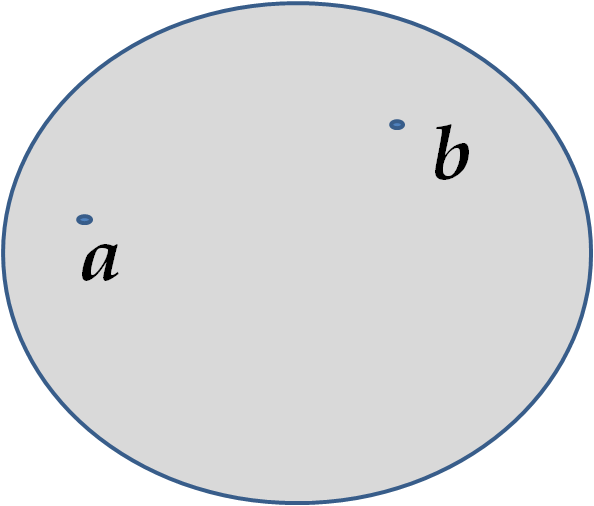Figure 32.3.3. Electric potential difference between two points in a conductor is zero. That is, all points of the the metal are at a single potential value. The outer surface is an equipotential surface. Here, $\phi_b = \phi_a\text{.}$

You can prove that $\phi_b = \phi_a$ by using Calculus. Since electric field inside is zero, we will easily do this.

\begin{align*} \phi_b-\phi_a \amp = - \int_a^b\vec E \cdot d\vec r\\ \amp = 0 \end{align*}

Therefore,

\begin{equation*} \phi_b = \phi_a, \end{equation*}

that is, a metal has the same potential everywhere when in electrostatic equilibrium.

Implications for Conductors with Cavities

If we put a charge $+q$ inside the cavity, then the charge separation takes place in the conductor as in Figure 32.3.4. This results in a total charge $-q$ on the inside surface and a total $+q$ amount of charge at the outside surface.

By using Gauss's law and the fact that electric field inside the conductor metal is zero, you can prove these facts. To prove that net charge at the inside surface of the cavity must total to $-q\text{,}$ draw a Gaussian surface in the metal. The flux through it must be zero since $E=0\text{.}$ Then, by adding up charges inside the Gaussian surface, you will conclude that the induced charge at the cavity is indeed $-q\text{.}$ Try a Gaussian surface that encloses all charges to show that at the oiutside surfac, the total charge induced is $+q\text{.}$

For the same conductor with a charge $+q$ outside the conductor, as in Figure 32.3.5, there will be no excess charge on the inside surface; both the positive and negative induced charges reside on the outside surface.Figure 32.3.5. A charge outside a conductor with a cavity. The cavity remains free of charge. The polarization of charges on the conductor happens at the outer surface of the conductor.

If there are two cavities in a conductor, with one of them having a charge $+q_a$ inside it and the other a charge $-q_b\text{,}$ the polarization of the conductor results in $-q_a$ on the inside surface of the cavity a, $+q_b$ on the inside surface of the cavity b, and $q_a-q_b$ on the outside surface (Figure 32.3.6). The charges on the surfaces may not be uniformly spread out - their spread depends upon the geometry; the only rule obeyed is that when the equilibrium has reached, the charge distribution in the conductor will be such that the electric field by the charge distribution in the conductor cancels the electric field of the external charges at all space points inside body of the conductor.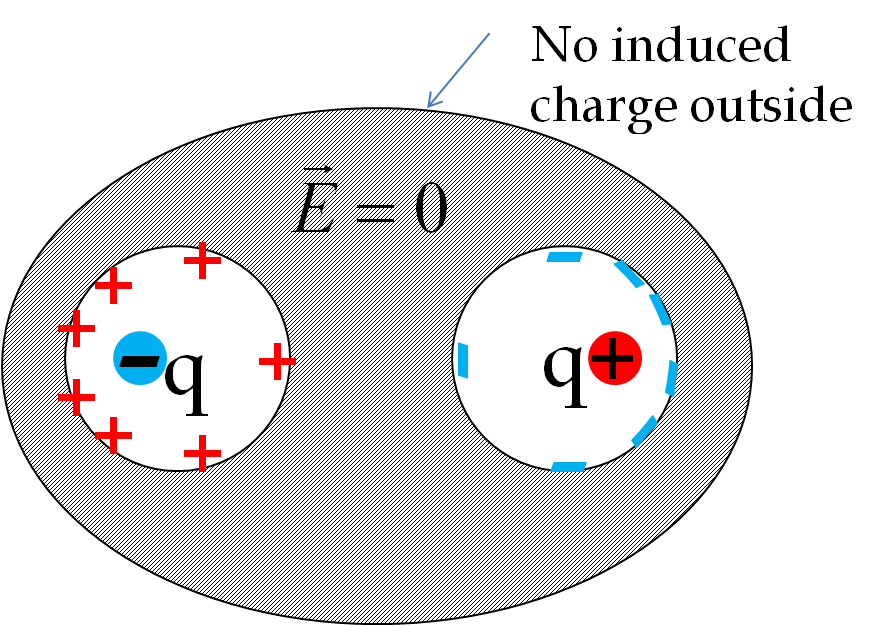Figure 32.3.6. The induced charges by two equal and opposite charges in two separate cavities of a conductor. If the net charge on the cavity were non-zero, the external surface will be charged to amount of the net charge.

Summary: Conductors in Electrostatics

We now summarize special aspects of electrostatics in conductors.

1. A metal has a large number of conduction electrons and conducts electricity by flow of these electrons. Other conductors have other mechanisms of flow of electricity through them.
2. No extra charges at a point in the volume. All extra charges on the surface, either inner or external surface, depending upon whether there are charges inside cavities of the material.
3. Electric field inside a conductor is zero in a static equilibrium.
4. A conductor has the same potential everywhere in its body. The surface of the conductor is an equipotential surface.

A negative charge $-q$ is fixed inside the cavity of a neutral spherical conductor as shown in Figure 32.3.8. (a) Show all induced charge distributions schematically, and draw electric field lines. (b) Draw equipotential lines on the same diagram using either a different color ink or as dashed lines.

Hint

Use the rule that: (1) Electric field lines begin at positive charge and end at negative charges and (2) Electric field lines land perpendicularly on metals.

See solution.

Solution

Answers for both (a) and (b) are shown in Figure 32.3.9. We use the rules given in the hint. Induced charges on the external surface are uniformly spread while the distribution at the inside surface of the cavity is not uniform. There is higher charge density at the cavity surface closer to the negative point charge located inside the cavity.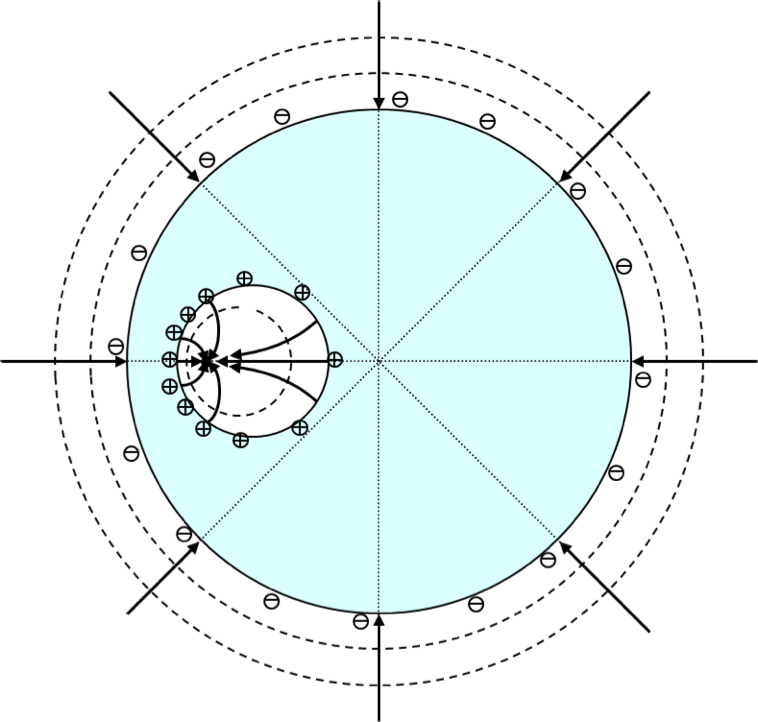Figure 32.3.9. Solution of Checkpoint 32.3.7. Here dashed lines are equipotential lines. In three-dimensions, these lines are actually closed surfaces.

An uncharged metallic ring is placed between two charged parallel plates of a parallel plate capacitor with charges $\pm Q$ as shown in Figure 32.3.11. Draw electric field lines and equipotential surfaces.

Hint

Use the rule that: (1) Electric field lines begin at positive charge and end at negative charges and (2) Electric field lines land perpendicularly on metals.